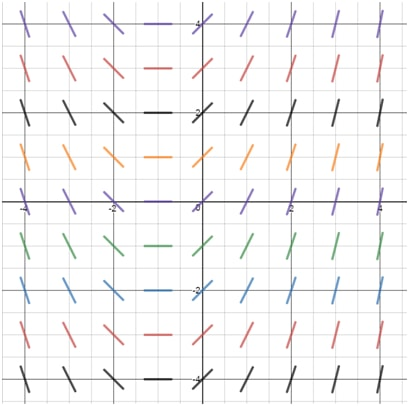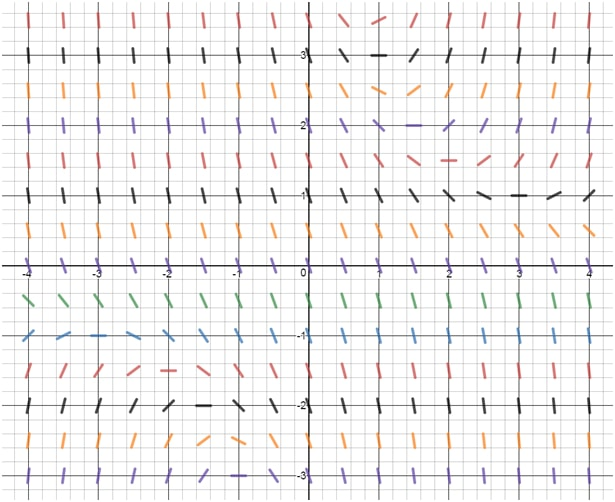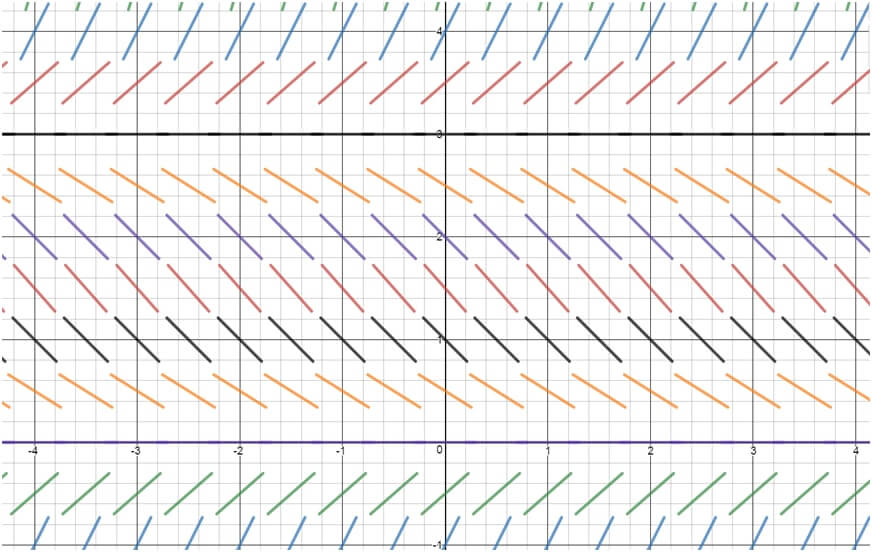# Slope fields#### Everything You Need in One Place

Homework problems? Exam preparation? Trying to grasp a concept or just brushing up the basics? Our extensive help & practice library have got you covered.#### Learn and Practice With Ease

Our proven video lessons ease you through problems quickly, and you get tonnes of friendly practice on questions that trip students up on tests and finals.#### Instant and Unlimited Help

Our personalized learning platform enables you to instantly find the exact walkthrough to your specific type of question. Activate unlimited help now!##### Intros
###### Lessons
1. What are Slope Fields?
##### Examples
###### Lessons
1. Understanding Slope Fields
Find the directional field for the following equations:
1. $\frac{dy}{dx}=xy-x$
2. $\frac{dy}{dx}=\frac{x^2}{(y+1)}$
2. Determining the Equation from a Slope Field
Which equation best corresponds to the following slope field?i.
$\frac{dy}{dx}=y-2$
ii.
$\frac{dy}{dx}=xy-2$
iii.
$\frac{dy}{dx}=x+1$
iv.
$\frac{dy}{dx}=-x+1$
1. Which equation best corresponds to the following slope field?i.
$\frac{dy}{dx}=xy-2$
ii.
$\frac{dy}{dx}=-\frac{y}{x}$
iii.
$\frac{dy}{dx}=2x+y$
iv.
$\frac{dy}{dx}=xy-3$
1. Given the differential equation and its resulting slope field:
$\frac{dy}{dx}=\frac{y}{2}(y-3)$Draw a solution to the following differential equation using the following initial value conditions:
1. $y(-2)=1$
2. $y(0)=4$
3. $y(1)=3$
###### Topic Notes
Slope fields, also called directional fields or vector fields, are graphical representations of first-order differential equations.

Slope Fields consist of a bunch of lines indicating the slope of y with respect to x, or $\frac{dy}{dx}$